# Calculus - Calculus MCQ

36:

The following function has a local manima at which value of x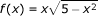A.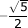B.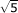C.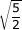D.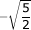Answer Report Discuss Option: C Explanation : Click on Discuss to view users comments. Write your comments here:
37:

If temperature field in a body varies according to the equation T(x, y)= x2 + 4xy, then direction of fastest variation in temperature at the point (1, 0) is given by

 A.B.C.D.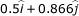Answer Report Discuss Option: C Explanation :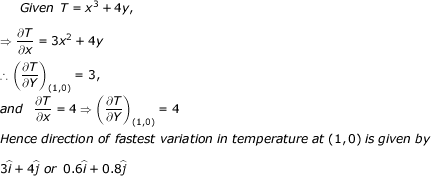Click on Discuss to view users comments. Write your comments here:
38:

If f(x) = 3x4 -4x2 +5, then the interval for which f(x) satisfied all the condition of Rolle's Theorem is

 A. [0, 2] B. [-1, 1] C. [-1,0] D. [1, 2] Answer Report Discuss Option: B Explanation :   Given function is, f(x) = 3x4 - 4x2 + 5 (i) f (x) is continuous in every real interval (ii) f ' (x) exist in any real interval (iii)  f(-1) = 3(-1)4 - 4(1)2 + 5 = 4        f(1) = 3(1)4 - 4(1)2 + 5 = 4                                f(-1) = f(1) Also            f ' (c) = 12c3 - 8c = 0  ⇒                     c = 0,c ∈ [-1, 1] Hence all the condition of Rolle's Theorem are satisfied in the interval [-1, 1]  Click on Discuss to view users comments. Write your comments here:
39:

The triangle of maximum area inscribed in a circle of radius r is

 A. a right angled triangle with hypotenuse measuring 2r B. an equilateral triangle C. an isosceles triangle of height r D. doesnot exist Answer Report Discuss Option: B Explanation :   Let ABC be a triangle inscribed in the circle with centre 0 and radius r. If area of this triangle is maximum, then vertex C should be at a maximum distance from the base AB i.e. , CD must be perpendicular to AB. Hence ABC is an isosceles triangle.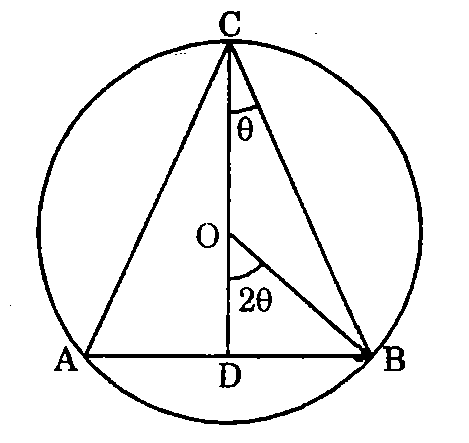If ∠ BCD = 0, where D is the mid.-point of BC, then                        ∠ BOD = 2ϑ  therefore,        AB= 2BD = 2r sin 2ϑ                         CD = CO + OD = r + r cos 2ϑ If S be the area of the triangle ABC, then                         S = 1/2 AB x CD = 1/2 x 2r sin 2 ϑ(r + r cos 2ϑ)  ds /dϑ   = r2 [sin 2ϑ (-2 sin 2ϑ) + (1 + cos 2ϑ) (2 cos 2ϑ)]             = 2r2 [cos 22ϑ -  sin2 2ϑ + cos 2ϑ] = 2r2 (cos 4ϑ + cos 2ϑ) For maximum and minimum, ds / dϑ = 0 cos 4ϑ + cos 2ϑ = 0 2 cos 3ϑ cos ϑ = 0  Hence either cos 3ϑ = 0, or cos ϑ = 0, which is impossible  if cos ϑ = 0,    then   ϑ = Π / 2 if  cos 3ϑ = 0, then 3 ϑ = Π / 2                                   ϑ  = Π / 6 ( a2s / dϑ 2) 6 = Π/6 is negative therefore    S is maximum for ϑ = 1 / 6 * Π                     ∠  ACB = 2ϑ = 2( Π / 6 ) = Π / 3 = ∠ ABC = ∠ BAC Hence ABC is an equilateral triangle. Click on Discuss to view users comments. Write your comments here:
40:

The greatest and least value of
f(x) = x4- 8x3 + 22x2 - 24x +1 in [0, 2] are

 A. 0, 8 B. 0, -8 C. 1,8 D. 1, -8 Answer Report Discuss Option: D Explanation : f(x) = x4- 8x3 + 22x2 - 24x +1 , f(0) = 1                                                    f(2) = 24 - 8.23 + 22.22 - 24.2 + 1 = -7 Now                                           f ' (x) = 4x3-24x2 + 44x - 24 For maximum and minimum,        f ' (x) = 0 ⇒                                                 4x3-24x2 + 44x - 24 = 0 ⇒                                                 4(x - 1)(x - 2)(x - 3) = 0 therefore                                      x = 1, 2, 3 Since x = 3 doesnot lie in [0, 2] therefore, consider only x = 1 and x = 2 We have                                     f(1) = 14 - 8.13 +22.12 - 24.1 + 1 = -8 Greatest of f(x) = largest of {1, -7, -8} = 1 Least of f(x) = smallest of {1, -7, -8} = -8 Click on Discuss to view users comments. Write your comments here: